#Function Repository Resource:

# LargestPrimeGap

Give the greatest gap between the primes up to the argument

Contributed by: Wolfram Staff
 ResourceFunction["LargestPrimeGap"][arg] gives the largest difference between primes up to arg, which must be at least 3.

## Examples

### Basic Examples

Here are the primes up to 13:

 In:=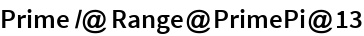Out=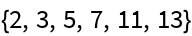The largest gap is between 7 and 11:

 In:=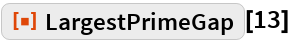Out=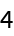The function is nondecreasing:

 In:=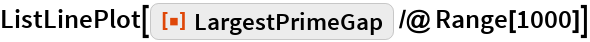Out=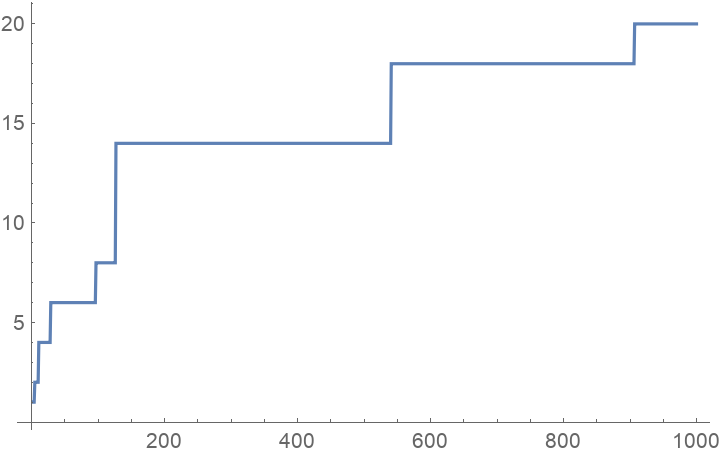Although the gaps are unbounded, they rise slowly:

 In:=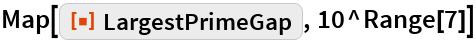Out=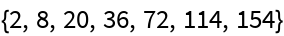## Requirements

Wolfram Language 11.3 (March 2018) or above